Courses
Courses for Kids
Free study material
Free LIVE classes
More

# Alternate Segment TheoremLIVE
Join Vedantu’s FREE Mastercalss

## Introduction to Alternate Segment Theorem

When a chord is drawn to a given circle, the region between the drawn chord and the corresponding arc is called a segment. Any chord drawn in a given circle always creates two segments, commonly known as major segment and minor segment.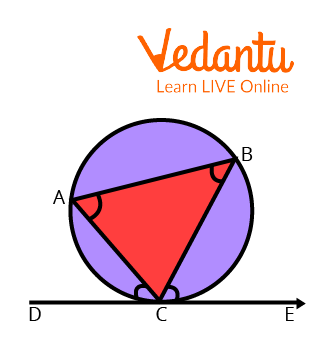A Circle

In the figure shown above, $DE$is a tangent to the circle with the point of contact at $C$, $BC$is a chord to the circle,$\angle BCE$ is the angle made between the chord $BC$and the tangent $DE$and $\angle CAB$ is the angle in the alternate major segment. The alternate segment theorem gives the relation between the angle made between a chord and the tangent made at one of the endpoints of the chord.

## Statement of Alternate Segment Theorem

For every circle, the angle created by the chord in the alternative segment equals the angle formed by the tangent via the point of contact of the tangent.

## Proof of the Alternate Segment Theorem

Given: $AB$ is a tangent to the given circle with the point of contact at $P$, $PQ$is a chord to the given circle and point $O$ represents the centre of the given circle.

To Prove: The angle formed between the chord and tangent in one segment of the circle is equal to the angle opposite to it in the alternate segment.

$\angle \alpha =\angle \beta$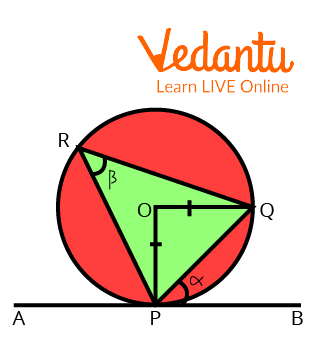Proof of the Alternate Segment Theorem

In the given figure, $AB$ is a tangent to the circle having a point of contact at $P$:

$\angle QPB = \alpha$ (Angle made by the tangent AB and the chord PQ)

$\angle PRQ = \beta$ (Angle made by the chord in the alternate segment)

As $\angle OPB = 90{}^\circ$ (Angle between tangent and radius of the circle)

$\angle OPQ = 90-\angle QPB$

$\angle OPQ = 90-\alpha$

$\angle OPQ = \angle OQP$( $\Delta OPQ$ is isosceles as $OP=OQ$)

$\angle POQ = 2\angle PRQ$(Angle subtended by a chord at the centre of a circle is twice the angle subtended at the circumference)

$\angle POQ = 2\beta$

$\angle OPQ + \angle OQP + \angle POQ = 180{}^\circ$(Angle sum property of a Triangle)

$2(90-\alpha ) + 2\beta = 180{}^\circ$

$2\alpha = 2\beta$

$\alpha = \beta$

Hence Proved

## Alternate Segment Theorem in Quadrilateral

Given: In the given figure $ABCD$ is a quadrilateral, and $ML$is a tangent to the circle having a point of contact at $A$, the chords of the circle are represented as $AB$, $AC$, and $AD$ respectively.

To prove: The alternate segment theorem is valid for quadrilaterals also, hence:

$\angle s = \angle q$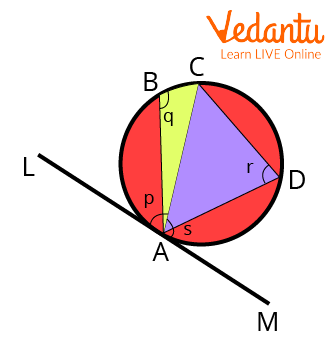According to Alternate Segment Theorem:

$\angle p = \angle r$

As the line $LM$is a straight line, so:

$\angle p + \angle s = 180{}^\circ$….. (1)

$\angle r$ and $\angle q$ are the opposite angles of a cyclic quadrilateral:

$\angle r + \angle q = 180{}^\circ$…… (2)

Comparing Equations (1) and (2):

$\angle p + \angle s = \angle r + \angle q$

We already have $\angle p = \angle r$

So, $\angle s = \angle q$

Hence Proved

## Application of Alternate Segment Theorem

• The alternate segment theorem can only be used for circles.

• The alternate segment theorem can only be used when there is a chord of the circle, a tangent is passing through one or both of the endpoints of the chord, and the angles that are used in the question are the angle between the chord and the tangent and the angle formed by the chord in the alternate segment.

• The alternate segment theorem helps to reduce a lot of large calculations and helps to reach an easy solution for the question.

### Important Points

• Tangent Chord theorem is the other name of the alternate segment theorem.

• The tangent of the given circle should pass through one of the endpoints of the given chord of the circle.

• Only that angle should be considered which the chord is forming in the alternate segment.

### Solved Examples

1. Calculate the value of angles x and y in the given diagram.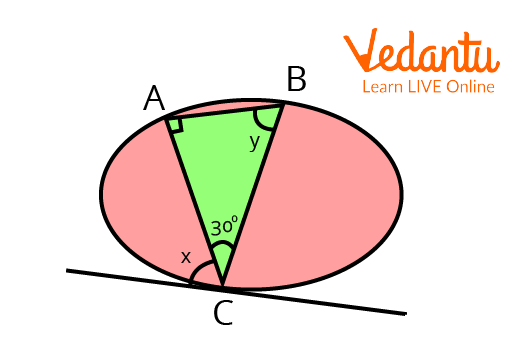Alternate Segment Theorem

Ans: The tangent to the circle has the point of contact at $C$, the angle formed between the tangent and the chord $AC$ is $\angle x$ and the chord $AC$ is subtending an $\angle y$ in the alternate segment of the circle.

$\angle x = \angle y$ (Alternate Segment Theorem)

$\angle ABC + \angle BAC + \angle ACB = 180{}^\circ$(Angle sum property of Triangle)

$\angle y+ 90 + 30 = 180$

$\angle y + 120 = 180$

$\angle y = 60{}^\circ$

2. Observe the figure and identify the angle that is not equal to $\angle SAD$?A Circle with 2 Triangles

Ans: Here, the $\angle SAD$ is the angle formed between the Tangent $ST$and the chord $AD$, and the chord $AD$is subtending two angles at the circumference of the circle in the alternate segment, which is $\angle ACD$and $\angle ABD$ respectively. Hence, according to the alternate segment theorem$\angle, ADB$is not equal to $\angle SAD$.

3.Find $X{}^\circ + Y{}^\circ$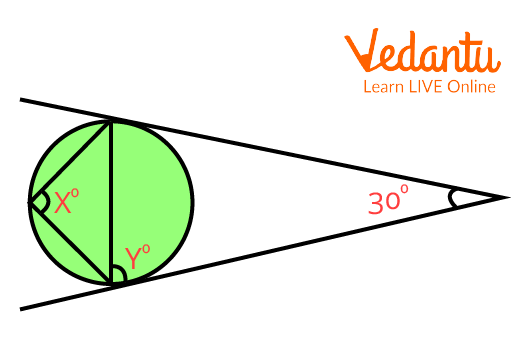A Circle with Two Unknown Angles

Ans: Here, $\angle Y$ is the angle between the chord and the tangent and $\angle X$ is the angle subtended at the circumference by the chord in the alternate segment.

Hence, $\angle X = \angle Y$

$\angle X$ and $30{}^\circ$are the opposite angles of a cyclic quadrilateral:

$\angle X + 30{}^\circ =180{}^\circ$

$\angle X = 150{}^\circ$

$\angle Y = 150{}^\circ$

## Conclusion

In this article, we discussed the Alternate Segment Theorem in depth. The proof, statement, applications, and examples are thoroughly explained above. From the discussion above about the alternate segment theorem, we can conclude that it is a very useful theorem to be used in solving questions of circles, and it helps us to reduce a lot of calculations.

Last updated date: 26th Sep 2023
Total views: 118.5k
Views today: 1.18k

## FAQs on Alternate Segment Theorem

1. Name some theorems other than alternate segment theorem which are used in problems related to circles?

In Class 9 and Class 10, students will come across the basics of circles. Some theorems topics are listed below:

Supplementary Angle Theorem-According to the supplementary angle theorem, if two angles are supplementary to the same angle, the two angles are said to be congruent.

The perpendicular from the Centre to a Chord splits the chord into two halves.

Length of an arc = $\dfrac{2\pi r\theta }{360}$

Cyclic Quadrilaterals – A type of Quadrilateral which is related to a circle in which the sum of opposite angles is 180o

2. What is the benefit of the alternate segment theorem?

The presence of an alternate segment theorem helps in the reduction of a lot of calculations. The student won’t have to use so many other theorems; otherwise, without an alternate segment theorem, we would have to use so many properties like the angle sum property of triangle, a cyclic quadrilateral theorem, and many more just to find the value of intermediate angles and then many more calculations will take place to get to the final answer.

3. What is meant by alternate segments?

When the chord of a circle is making one angle with the tangent of a circle, and it is subtending another angle at the circumference of the circle, then the segments in which both these angles lie are referred to as alternate segments. A quite easy way to define alternate segments would be when all the calculations are taking place in one segment of a circle, and one angle is lying in the other segment of the circle, then we will say that that angle is lying in the alternate segment.